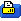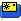This thread is privately moderated by Nereth, who may elect to delete unwanted replies.
Aug 12, 2013, 06:50 AM
Registered User
Discussion

# Some mathematical relationships for predicting the effect of power system changes.

Just some simple formulas that dont take into account much by way of real world phenomenon like losses, but they can be useful for small changes:

Thrust:

1) Thrust from increased RPM, changes ∝ n^2
1) Thrust from Increased average speed due to diameter, changes ∝ D^2
2) Thrust from Increased length due to diameter, changes ∝ D
3) Thrust from Increased Chord, (not sure the symbol but calling it C) changes ∝ C. We assume this is proportional to diameter, therefore changes ∝ D.
4) Thrust from decreased average AOA, changes ∝ D^-1
5) Thrust from increased pitch, changes ∝ P.

So in total we get this:

Thrust ∝ N^2*D^2*D*D*D^-1*P = N^2*D^3*P

Current

Current is linear with torque, torque linear with thrust, so by the same token:

Current ∝ N^2*D^3*P

For 0 resistance or inductance,

N ∝ V
and also,
N ∝ Kv

Therefore,

N ∝ V*Kv
 Sign up now to remove ads between postsThread ToolsShow Printable VersionEmail this Page